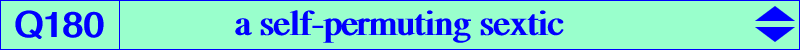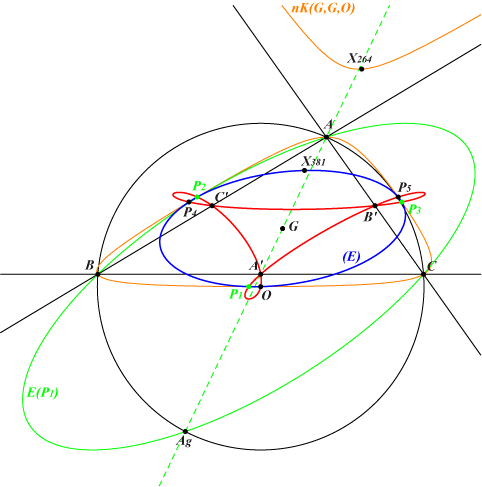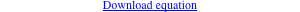too complicated to be written here. Click on the link to download a text file.X(3) midpoints of ABC (double points) infinite points of the sidelines of ABC (quadruple points) permutations of O, see belowQ180 answers a question raised by Dan Reznik. Q180 is the locus of centers P of circum-ellipses E(P) whose area is that of the circumcircle (O). Recall that a circum-conic with center P is an ellipse if and only if P lies in one of the four regions bounded by the sidelines of the medial triangle and containing G or one vertex of the antimedial triangle. With T1 = a + b + c, T2 = a b + b c + c a, T3 = a b c, its equation takes the form : T1^3 (T1^3 - 4 T1 T2 + 8 T3)^3 x^2 y^2 z^2 + T3^4 (-x+y+z) (x-y+z) (x+y-z) (x+y+z)^3 = 0. For every P = x:y:z on the curve, five other points P1, P2, P3, P4, P5, obtained under all permutations of the coordinates of P, lie on the curve, although not necessarily all distinct. This is obvious from the equation above. P1 = x:z:y, P2 = z:y:x, P3 = y:x:z, are the points obtained when two coordinates are swapped. P4 = z:x:y and P5 = y:z:x are the remaining points. These are bicentric points such as the Brocard points when P is a triangle center. It is known that the six points lie on a same ellipse (E) with center G, homothetic to the Steiner ellipse. They also lie on a Tucker cubic nK(G, G, P). See further details here. Note that these cubics are also self-permuting curves. Q180 is therefore invariant under the three oblique symmetries whose axes are the medians and directions the relative sidelines of ABC. *** In particular, with P = O, we obtain the points on the figure above. P1 = a^2 SA : c^2 SC : b^2 SB = sin 2A : sin 2C : sin 2B. P2 and P3 are obtained similarly. The G-Ceva conjugate G/P1 of P1 is a^2 : c^2 : b^2, the A-vertex of the first Brocard triangle. P1, P2, P3 are the isogonal conjugates of Oa, Ob, Oc. These points are the centers of the polar conics of A, B, C in K006. More details there. {P4, P5} is the bicentric pair PU(38). {G/P4, G/P5} is the bicentric pair PU(11) formed by the isotomic conjugates of the Brocard points Ω1, Ω2. P4 and P5 are the common points of the line {5, 525} and the circum-conic with perspector X(16089), the G-Hirst inverse of X(264).